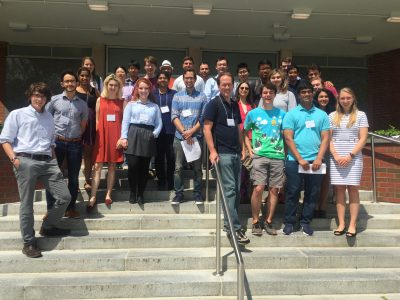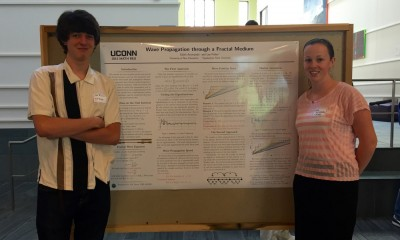# 5th Northeast Mathematics Undergraduate Research Mini-Symposium

### University of Connecticut, August 3rd, 2017

#### Participating Schools: Amherst, Smith, UConn and UMass

5th Mini-Symposium full program (2017)## Overview

Laplacians have been well studied on post-critically finite (PCF) fractals. However, less is known about gradients on such fractals. Building on work by Teplyaev, we generalize results regarding the existence and continuity of the gradient on the standard Sierpinski Gasket to higher dimensional Sierpinski Gaskets. In particular, we find that, for functions with a continuous Laplacian, the gradient must be defined almost everywhere, and specify a set of points for which it is defined. Furthermore, we provide a counterexample on higher-dimensional Sierpinski gaskets where the Laplacian is continuous but the gradient is not defined everywhere. We conjecture that Hölder continuity of the Laplacian is a condition strong enough to guarantee that the gradient exists at each point.

# Spectral Analysis on Graphs Related to the Basilica Julia Set

## Overview

We analyze the spectra of a sequence of graphs constructed from the Schreier graphs of the Basilica group.  Our analysis differs from earlier work of Grigorchuk and Zuk in that it is based on a macroscopic decomposition of the graphs. This method gives precise information about the multiplicities of eigenvalues and, consequently, good information about the spectral measures of large graphs. It also permits a proof of the existence of gaps in the spectrum of limiting graphs.

# Spectrum of the Magnetic Laplacian on the Diamond Fractal

## Overview

The diamond fractal is a fractal that is obtained in the following manner.  Start with a graph with two vertices and an edge and replace the edge with two new vertices connected to our original vertices to obtain a diamond shaped graph.   The diamond fractal is defined to be the limiting object after continuing with the edge replacement indefinitely.  In the project, the spectrum of magnetic Laplacian operators on graph approximations to the diamond fractal was computed.

Given a level n approximation to the fractal with known magnetic field strengths through cells and holes, it is possible to determine the net magnetic field through the cells and holes of the preceding graph approximations.  The spectral similarity relation between the operators on successive graph approximations was worked out, with the corresponding spectral decimation polynomial depending on the magnetic field strengths.  A poster and talk on this work was presented at the REU Mini-Symposium at UConn.

# arXiv:1704.01609

## Presentation

Magnetic Spectral Decimation

Poster

# Publication

Paper

Joe P Chen8,1, Luke G Rogers9,2, Loren Anderson3, Ulysses Andrews2, Antoni Brzoska2, Aubrey Coffey4, Hannah Davis3, Lee Fisher5, Madeline Hansalik6, Stephen Loew7Show full author list

Published 14 July 2017 © 2017 IOP Publishing Ltd
, , Number 32 Citation Joe P Chen et al 2017 J. Phys. A: Math. Theor. 50 325205

arXiv.org

## Project

Project Information

# Power Dissipation in Fractal AC Circuits

## Supervisors

Alexander TeplyaevLuke Rogers, Antoni Brzoska, Ulysses Andrews

## Overview

In this project, Feynman’s analysis of an infinite ladder circuit is extended to fractal circuits, in particular, a Sierpinski Ladder circuit and two variants of a Hanoi circuit.   These circuits are constructed by taking the limit of graph approximations with inductors and capacitors placed along edges in a well-defined manner.  Inductors, capacitors and resistors all contribute to the impedance within a circuit; but whereas a resistor imparts a real impedance, inductors and capacitors impart a purely imaginary impedance.

For each circuit, the following was accomplished.  First, the net impedance between certain boundary points of the circuit was computed.  Second, the filter conditions for each circuit were found.  A circuit becomes a filter when the net impedance between boundary vertices has a positive real part!  Third, it was proved that these impedances can be obtained by placing a small positive resistance epsilon on each edge of the graph approximations, finding the limiting impedance between the boundary vertices, and then taking epsilon to zero.  Finally, the construction of harmonic functions on these circuits was outlined.

# arXiv:1605.03890

## Presentation

Fractal AC Circuits

Poster

# Group Members

Edith Aromando, Lee Fisher## Overview

We consider the wave equation on the unit interval with fractal measure, and use two numerical models to study wave speed and propagation distance.  The first approach uses a Fourier series of eigenfunctions of the fractal Laplacian, while the second uses a Markov chain to model the transmission and reflection of classical waves on an approximation of the fractal. These models have complementary advantages and limitations, and we conjecture that they approximate the same fractal wave.

Poster